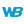• Coordinates
• Drawing in PHP
• Drawing in Browser code
WINDEVWEBDEVWINDEV MobileOthers<Image variable>.DrawLine (Function)
In french: <Variable Image>.DessineLigne
Draws a line in a variable of type Image.Caution: A specific configuration is required to use this function in Linux. For more details, see The drawings.
Example
MyImage is Image = "Test.gif"
// Draw a light yellow line in an Image variable
MyImage.DrawLine(10, 10, 50, 50, LightYellow)
IMG_MyDrawing = MyImage
Syntax
<Image variable>.DrawLine(<X1> , <Y1> , <X2> , <Y2> [, <Line color> [, <Line thickness>]])
<Image variable>: Image variable
Name of the Image variable to be used.
<X1>: Integer
X-coordinate (in pixels) of the start point of the line.
<Y1>: Integer
Y-coordinate (in pixels) of the start point of the line.
<X2>: Integer
X-coordinate (in pixels) of the end point of the line.
<Y2>: Integer
Y-coordinate (in pixels) of the end point of the line.
<Line color>: Integer or constant (optional)
Line color. This color can correspond to:
• an RGB color (returned by RGB),
• an HSL color (returned by HSL),
• a WLanguage preset color,
•a variable of type Color.
If this parameter is not specified, the line color:
<Line thickness>: Optional integer
Thickness (in pixels) of the line to draw. If this parameter is not specified, the value taken into account can be:
Remarks

CoordinatesCoordinates are specified with respect to the upper-left corner of the image or Image control (coordinates: (0,0)).
Related Examples:Unit examples (WINDEV): The drawing functions [ + ] Using the main drawing functions of WINDEV to:- Initialize an Image control for drawing- Draw simple shapes- Write a text into a drawing- Change the color in a drawing
Business / UI classification: Neutral code
Component: wd280pnt.dll
Minimum version required
• Version 24